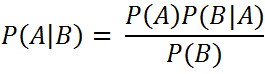`  I request GUEST WRITERS for this web site. Anyone interested please contact me at saravu2k2013@gmail.com Thanks  `

## Lossless Join Decomposition

Question:

Let R = {ssn, ename, pnumber, pname, plocation, hours} and R is decomposed into three relations R1, R2, and R3 as follows;
R1 = EMP = {ssn, ename}
R2 = PROJ = {pnumber, pname, plocation}
R3 = WORKS_ON = {ssn, pnumber, hours}
Assume that the following functional dependencies are holding on relation R.
F = {ssn → ename; pnumber → {pname, plocation}; {ssn, pnumber} → hours}.
Find whether the decomposition into R1, R2, and R3 is lossless join decomposition or not.

In theory, if a relation R is decomposed into relations R1 and R2 then the decomposition is lossless if either of the following holds;

• (R1 ∩ R2) → R1
• (R1 ∩ R2) → R2
In our problem, if we apply intersection between R1 and R2, we shall get nothing, that is, no attribute is common between R1 and R2.
Hence, let us apply intersection between R1 and R3. Now we shall get ssn as result.
(R1 ∩ R3) ({ssn, ename} ∩ {ssn, pnumber, hours}) {ssn}.
From the given set of functional dependencies F, we understand that, ssn → ssn, ename. That is,
({ssn, ename} ∩ {ssn, pnumber, hours}) → {ssn, ename} {ssn} → {ssn, ename} (R1 ∩ R3) → R1.
Hence, the decomposition into R1 and R3 is lossless.

Similarly, the decomposition into R2 and R3 is also lossless.
({pnumber, pname, plocation} ∩ {ssn, pnumber, hours}) → {pnumber, pname, plocation} (R2 ∩ R3) → R2.

So, we can conclude that decomposition of R into R1, R2, and R3 is lossless join decomposition.

********

Normalization solved examples
normalization exercises solved
what is lossless decomposition
rules for lossless join decomposition
lossless decomposition example
how to find whether a decomposition is lossless or not
lossless join decomposition one more example